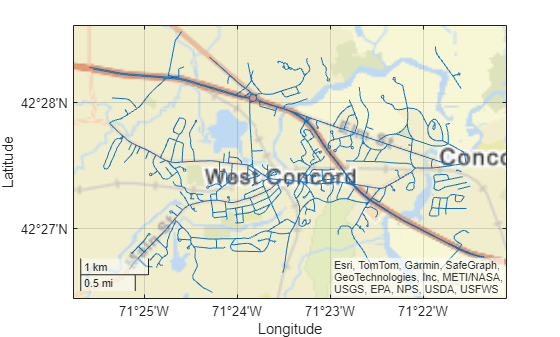# projinv

Unproject x-y map coordinates to latitude-longitude coordinates

## Syntax

``````[lat,lon] = projinv(proj,x,y)``````

## Description

example

``````[lat,lon] = projinv(proj,x,y)``` transforms the map coordinates specified by `x` and `y` in the projected coordinate reference system specified by `proj` to the latitude-longitude coordinates `lat` and `lon`. Specify `proj` using a `projcrs` object (since R2020b), a map projection structure, or a GeoTIFF information structure.```

## Examples

collapse all

Unproject x-y coordinates to latitude-longitude coordinates by specifying the projected CRS of the x-y coordinates. Then, display the latitude-longitude coordinates on geographic axes.

To do this, first import a shapefile containing the x- and y-coordinates of roads in Concord, MA. Get information about the shapefile as a structure. Find the projected CRS for the coordinates by accessing the `CoordinateReferenceSystem` field of the structure.

```roads = shaperead('concord_roads.shp'); x = [roads.X]; y = [roads.Y]; info = shapeinfo('concord_roads.shp'); proj = info.CoordinateReferenceSystem;```

Unproject the x-y coordinates to latitude-longitude coordinates.

`[lat,lon] = projinv(proj,x,y);`

Display the coordinates on geographic axes.

```figure geoplot(lat,lon) hold on geobasemap('streets')```The geographic CRS of the x-y coordinates used in this example is NAD83. You can find the geographic CRS that underlies a projected CRS by querying the `GeographicCRS` property.

`proj.GeographicCRS.Name`
```ans = "NAD83" ```

The geographic CRS underlying the `'streets'` basemap is WGS84. NAD83 and WGS84 are similar, but not identical. Therefore, at high zoom levels the coordinates and basemap may appear misaligned.

## Input Arguments

collapse all

Map projection, specified as a `projcrs` object (since R2020b), a scalar map projection structure (`mstruct`), or a GeoTIFF information structure. For more information about map projection structures, see `defaultm`. For more information about GeoTIFF information structures, see `geotiffinfo`.

Data Types: `struct`

Projected x-coordinates, specified as a scalar value, vector, matrix, or N-D array. The size of `x` and `y` must match.

Data Types: `single` | `double`

Projected y-coordinates, specified as a scalar value, vector, matrix, or N-D array. The size of `x` and `y` must match.

Data Types: `single` | `double`

## Output Arguments

collapse all

Geodetic latitudes, returned as a scalar value, vector, matrix, or N-D array, in units of degrees.

The geographic CRS of `lat` matches the geographic CRS of `proj`. If `proj` is a `projcrs` object, then you can find its geographic CRS by querying its `GeographicCRS` property. For example, this code shows how to create a `projcrs` object from EPSG code 32610 and find the associated geographic CRS.

```proj = projcrs(32610); proj.GeographicCRS.Name```
```ans = "WGS 84"```

Geodetic longitudes, returned as a scalar value, vector, matrix, or N-D array, in units of degrees.

The geographic CRS of `lat` matches the geographic CRS of `proj`. If `proj` is a `projcrs` object, then you can find its geographic CRS by querying its `GeographicCRS` property. For example, this code shows how to create a `projcrs` object from EPSG code 32610 and find the associated geographic CRS.

```proj = projcrs(32610); proj.GeographicCRS.Name```
```ans = "WGS 84"```

## Version History

Introduced before R2006a

expand all